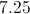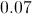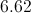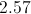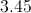# High School Math : Basic Statistics

## Example Questions

1 2 3 5 Next →

### Example Question #1 : How To Find The Standard Deviation For A Set Of Data

A bird watcher observed how many birds came to her bird feeder for four days.  These were the results:

Day 1: 15

Day 2: 12

Day 3: 10

Day 4: 13

Which answer is closest to the standard deviation of the number of birds to visit the bird feeder over the four days?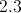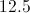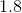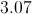Explanation:

Standard deviation is essentially the average distance from the mean of a group of numbers.  There are a number of steps in computing standard deviation, but the steps are not too complicated if you take them one at a time.  First, find the mean of the values.  Second, subtract the mean from the first value and square the result.  Do this for all remaining values.  Third, add these results together.  Fourth, divide this value by the number of values.  Finally, find the square root of the result.

1: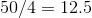2: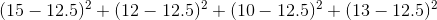3: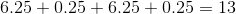4: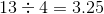5: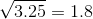### Example Question #2 : How To Find The Standard Deviation For A Set Of Data

Alice recorded the outside temperature at noon each day for one week. These were the results.

Monday: 78

Tuesday: 85

Wednesday: 82

Thursday: 84

Friday: 82

Saturday: 79

Sunday: 80

What is the standard deviation of the temperatures?